# RD Sharma Class 9 Solutions Chapter 1 Number Systems Ex 1.2

## RD Sharma Class 9 Solutions Chapter 1 Number Systems Ex 1.2

These Solutions are part of RD Sharma Class 9 Solutions. Here we have given RD Sharma Class 9 Solutions Chapter 1 Number Systems Ex 1.2

Question 1.
Express the following rational numbers as decimals: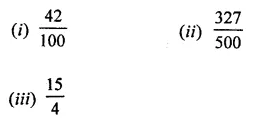Solution: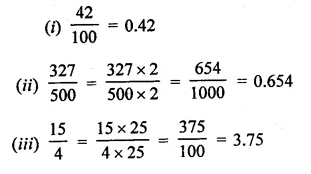Question 2.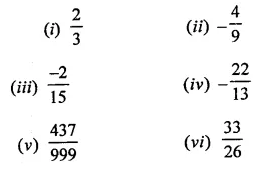Solution: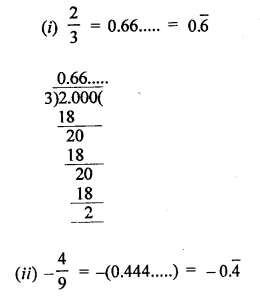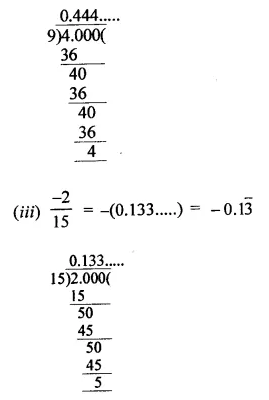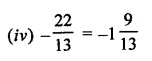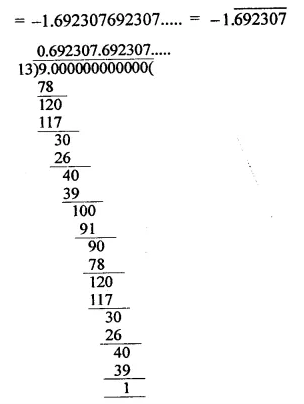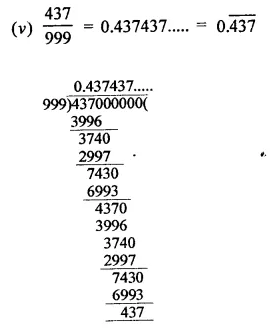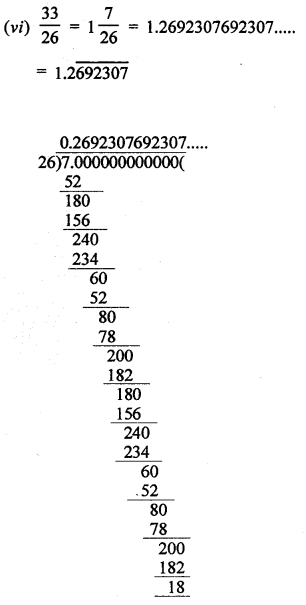Question 3.
Look at several examples of rational numbers in the form $$\frac { p }{ q }$$ (q ≠ 0), where p and q are integers with no common factors other than 1 and having terminating decimal representations. Can you guess what property q must satisfy?
Solution:
The property is if the denominators have factors 2 or 5 or both, the decimal representation will be terminating e.g.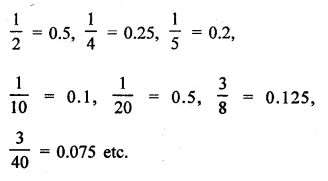Hope given RD Sharma Class 9 Solutions Chapter 1 Number Systems Ex 1.2 are helpful to complete your math homework.

If you have any doubts, please comment below. Learn Insta try to provide online math tutoring for you.